# 最受技术人员关心的四个「以太坊2.0 Staking」硬核问题

prysm 的实现主要包含两部分: 信标链客户端和验证者客户端。前者负责信标链的状态管理，后者负责验证者的出块和⻅证。为方便这一流程，prysm 提供了简易脚本 prysm.sh 来下载安装：

``mkdir prysm && cd prysm   curl https://raw.githubusercontent.com/prysmaticlabs/prysm/master/prysm.sh --output prysm.sh && chmod +x prysm.sh``

（向左滑动，查看完整代码）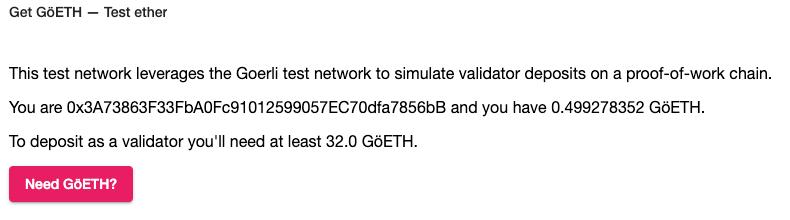./prysm.sh validator accounts create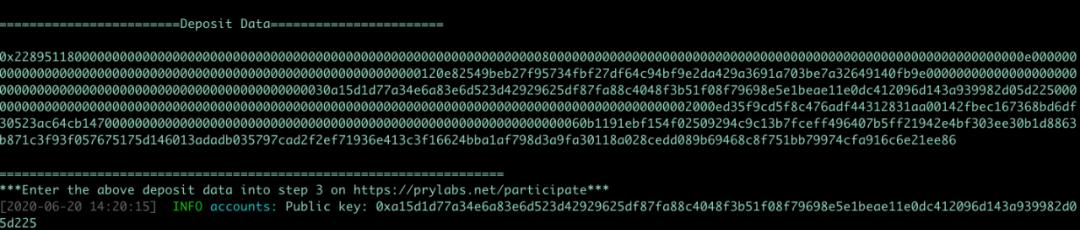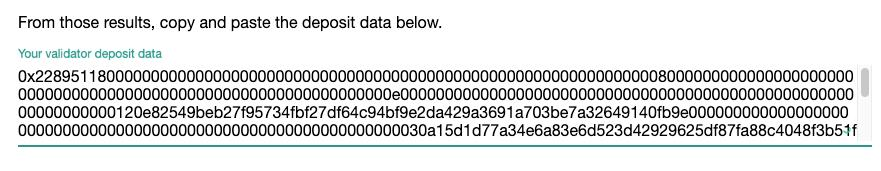``./prysm.sh beacon-chain./prysm.sh validator``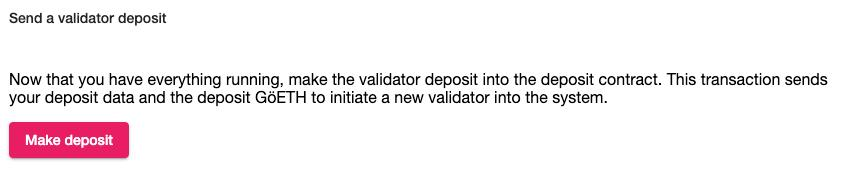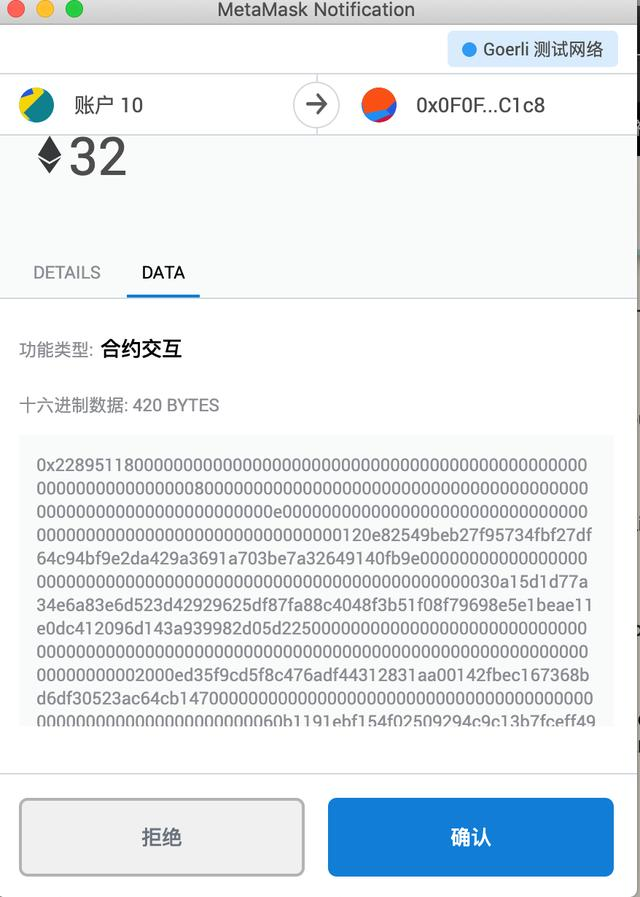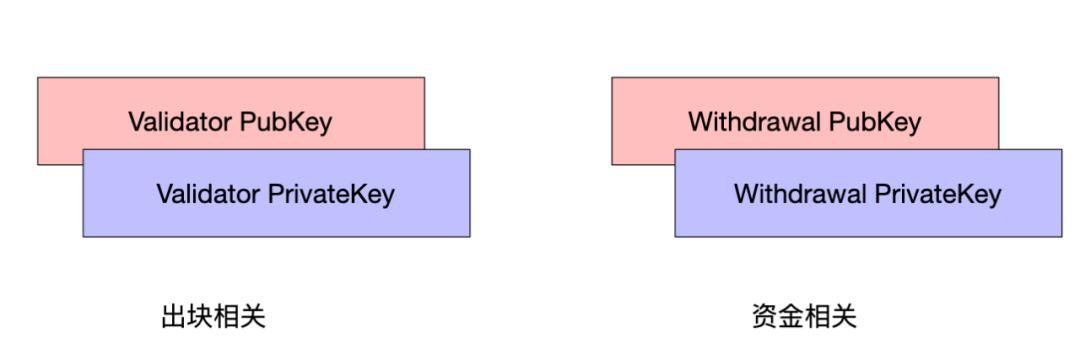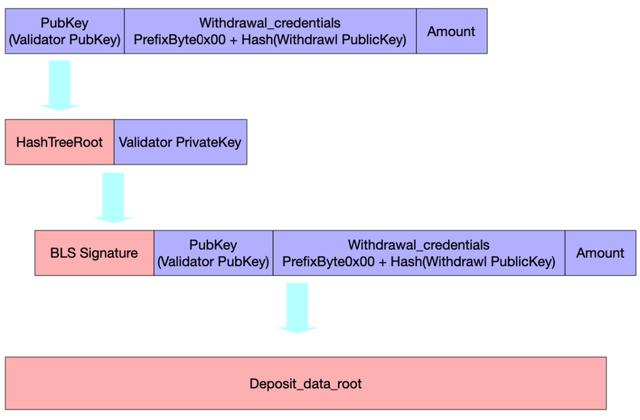• pubkey 是 Validator PubKey，这也是创建的验证者的公钥标识
• withdrawal_crendentials由一个固定前缀拼接 Withdrawal PubKey 的哈希（sha256）构成
• amount 是本次合约发送的金额，至少为1 ETH
• signature 是采用 Validator PrivateKey对pubkey、withdrawal_credentials、amount 的哈希（HashTreeRoot）结果的签名
• deposit_data_root 是前面4个参数的哈希（HashTreeRoot）

deposit 方法接受 pubkey（验证者公钥）、withdrawal_credentials（提取存款权限信息），signature（签名）和 deposit_data_root（防止篡改标识）作为参数。主要分成参数基础校验、触发存款事件、检验数据完整性、更新数据结构几个部分，如以下代码所示：

``  @public    def deposit(pubkey: bytes[PUBKEY_LENGTH],                withdrawal_credentials: bytes[WITHDRAWAL_CREDENTIALS_LENGTH],                signature: bytes[SIGNATURE_LENGTH],                deposit_data_root: bytes32):            ############## 1. 参数基础校验 ################        # Avoid overflowing the Merkle tree (and prevent edge case in computing `self.branch`)        assert self.deposit_count           # Check deposit amount        deposit_amount: uint256 = msg.value / as_wei_value(1, "gwei")        assert deposit_amount >= MIN_DEPOSIT_AMOUNT            # Length checks for safety        assert len(pubkey) == PUBKEY_LENGTH        assert len(withdrawal_credentials) == WITHDRAWAL_CREDENTIALS_LENGTH        assert len(signature) == SIGNATURE_LENGTH        #########################################            # Emit `DepositEvent` log        amount: bytes = self.to_little_endian_64(deposit_amount)                    ############## 2. 触发存款事件 ################        log.DepositEvent(pubkey, withdrawal_credentials, amount, signature, self.to_little_endian_64(self.deposit_count))                    ############## 3. 校验数据完整性 ################        # Compute deposit data root (`DepositData` hash tree root)        zero_bytes32: bytes32 = 0x0000000000000000000000000000000000000000000000000000000000000000        pubkey_root: bytes32 = sha256(concat(pubkey, slice(zero_bytes32, start=0, len=64 - PUBKEY_LENGTH)))        signature_root: bytes32 = sha256(concat(            sha256(slice(signature, start=0, len=64)),            sha256(concat(slice(signature, start=64, len=SIGNATURE_LENGTH - 64), zero_bytes32)),        ))        node: bytes32 = sha256(concat(            sha256(concat(pubkey_root, withdrawal_credentials)),            sha256(concat(amount, slice(zero_bytes32, start=0, len=32 - AMOUNT_LENGTH), signature_root)),        ))        # Verify computed and expected deposit data roots match        assert node == deposit_data_root        ###########################################                    ############## 4. 更新Merkle Tree ################        # Add deposit data root to Merkle tree (update a single `branch` node)        self.deposit_count += 1        size: uint256 = self.deposit_count        for height in range(DEPOSIT_CONTRACT_TREE_DEPTH):            if bitwise_and(size, 1) == 1:  # More gas efficient than `size % 2 == 1`                self.branch[height] = node                break            node = sha256(concat(self.branch[height], node))            size /= 2 ``

（向左滑动，查看完整代码）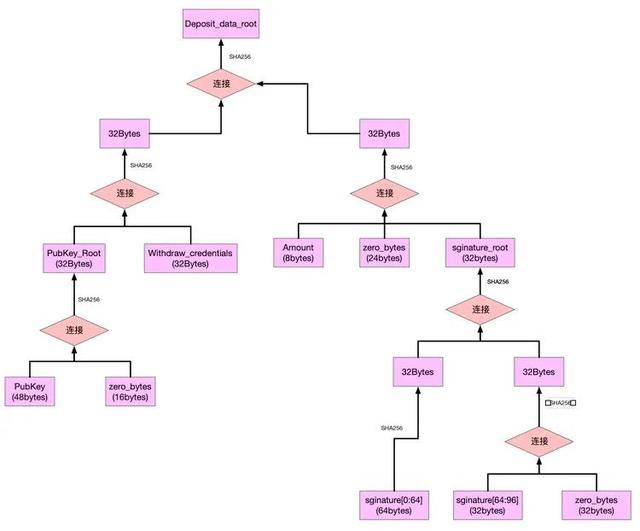``  def process_deposit(state: BeaconState, deposit: Deposit) -> None:            ##################### 必要检验 ###################        # Verify the Merkle branch        assert is_valid_merkle_branch(            leaf=hash_tree_root(deposit.data),            branch=deposit.proof,            depth=DEPOSIT_CONTRACT_TREE_DEPTH + 1,  # Add 1 for the List length mix-in            index=state.eth1_deposit_index,            root=state.eth1_data.deposit_root,        )            # Deposits must be processed in order        state.eth1_deposit_index += 1            pubkey = deposit.data.pubkey        amount = deposit.data.amount        validator_pubkeys = [v.pubkey for v in state.validators]        if pubkey not in validator_pubkeys:            # Verify the deposit signature (proof of possession) which is not checked by the deposit contract            deposit_message = DepositMessage(                pubkey=deposit.data.pubkey,                withdrawal_credentials=deposit.data.withdrawal_credentials,                amount=deposit.data.amount,            )            domain = compute_domain(DOMAIN_DEPOSIT)  # Fork-agnostic domain since deposits are valid across forks            signing_root = compute_signing_root(deposit_message, domain)            if not bls.Verify(pubkey, signing_root, deposit.data.signature):                return        #################################################                ##################### 添加新验证人 ###################            # Add validator and balance entries            state.validators.append(get_validator_from_deposit(state, deposit))            state.balances.append(amount)        #################################################        else:            ################ 同一个验证人多次存入 ###############            # Increase balance by deposit amount            index = ValidatorIndex(validator_pubkeys.index(pubkey))            increase_balance(state, index, amount)        ################################################# ``

（向左滑动，查看完整代码）

``````def get_validator_from_deposit(state: BeaconState, deposit: Deposit) -> Validator:      amount = deposit.data.amount
# 设置有效余额 effective_balance = min(amount - amount % EFFECTIVE_BALANCE_INCREMENT, MAX_EFFECTIVE_BALANCE)
# 设置验证者信息和生命周期相关参数 return Validator( pubkey=deposit.data.pubkey, withdrawal_credentials=deposit.data.withdrawal_credentials, activation_eligibility_epoch=FAR_FUTURE_EPOCH, activation_epoch=FAR_FUTURE_EPOCH, exit_epoch=FAR_FUTURE_EPOCH, withdrawable_epoch=FAR_FUTURE_EPOCH, effective_balance=effective_balance, )``````

（向左滑动，查看完整代码）

``  def process_registry_updates(state: BeaconState) -> None:        # Process activation eligibility and ejections        for index, validator in enumerate(state.validators):                # 可否进入等待队列            if is_eligible_for_activation_queue(validator):                validator.activation_eligibility_epoch = get_current_epoch(state) + 1                # 可否成为活跃的验证者            if is_active_validator(validator, get_current_epoch(state)) and validator.effective_balance               initiate_validator_exit(state, ValidatorIndex(index))            # 限制每周期成为验证者数量        # Queue validators eligible for activation and not yet dequeued for activation  15.      activation_queue = sorted([            index for index, validator in enumerate(state.validators)            if is_eligible_for_activation(state, validator)            # Order by the sequence of activation_eligibility_epoch setting and then index        ], key=lambda index: (state.validators[index].activation_eligibility_epoch, index))        # Dequeued validators for activation up to churn limit        for index in activation_queue[:get_validator_churn_limit(state)]:            validator = state.validators[index]            validator.activation_epoch = compute_activation_exit_epoch(get_current_epoch(state)) ``

（向左滑动，查看完整代码）

``      [m / 0] - [m / 0 / 0]     /        \    /           [m / 0 / 1][m] - [m / 1]    \     ...      [m / i]``

HashTreeRoot 提供了将一个对象按一定格式构建默克尔树、并求得树的默克尔根值的方法。HashTreeRoot 可以将一个对象（bit、bytes、vector、list、containers 等等）的各个部分按序排列然后构建默克尔树，获得根值。具体规范参考：https://github.com/ethereum/eth2.0-specs/blob/dev/ssz/simple-serialize.md

• 对pubkey、withdrawal_credentials、amount、signature 进行 HashTreeRoot，求得 deposit_data_root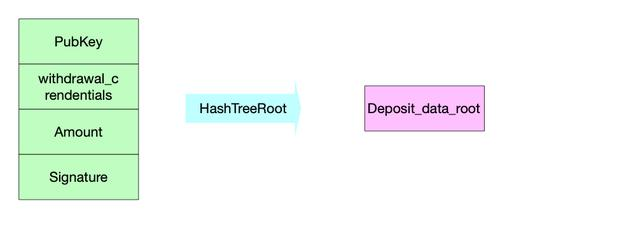• 对于整体的 HashTreeRoot，实际上是对各个部分分别求取 HashTreeRoot，最后一起 Merkleize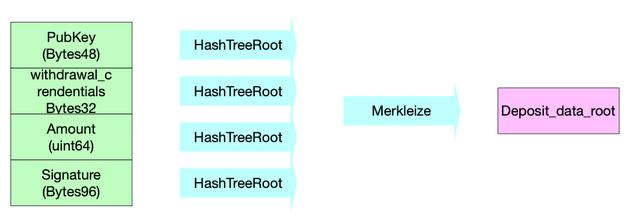• 每个部分的 HashTreeRoot 实际上是先 Pack 再将结果 Merkleize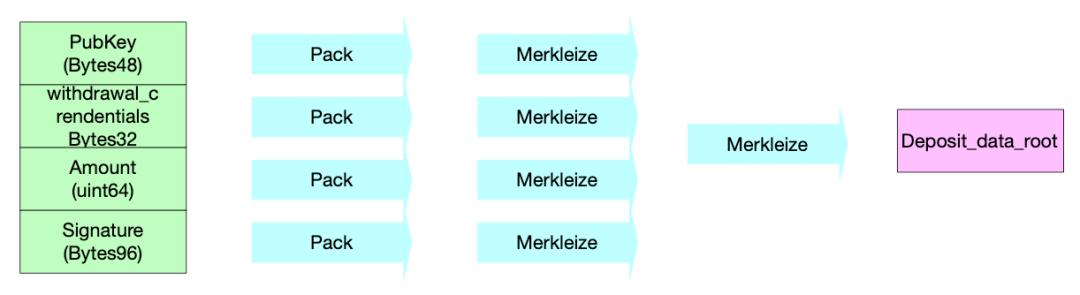• 如何进行 Pack？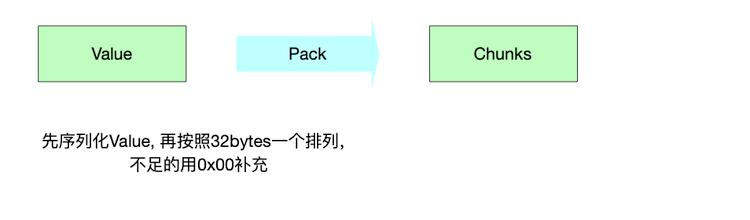• 如何进行 Merkleize？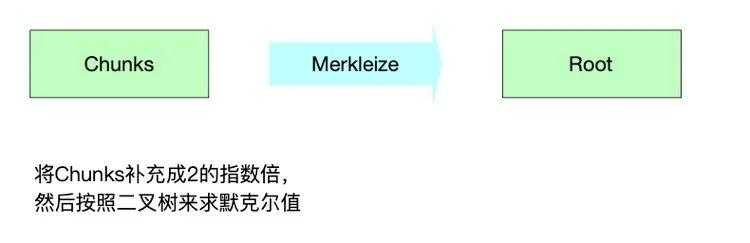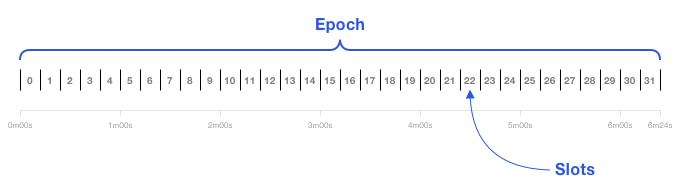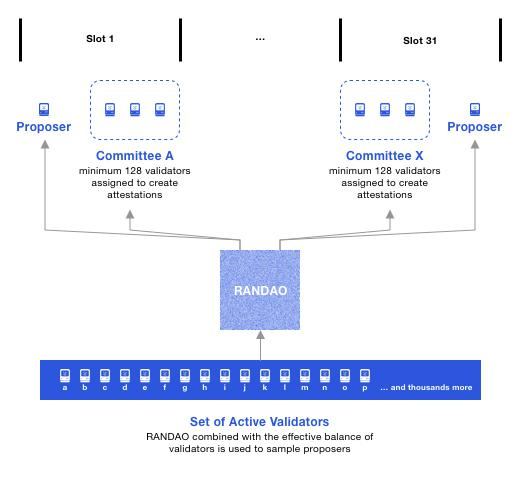⻅证消息（Attestation）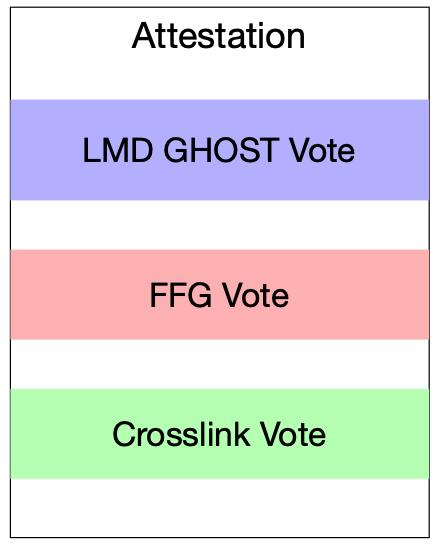• 使用 LMD Ghost 分叉算法，选出主链。
• 使用 Casper FFG 进行检查点（Checkpoint）的敲定。
• 对分片链状态（Crosslink）的投票（在 Phase 0 阶段还没有实现)）

LMD GHOST和Casper FFG

• 无利害关系（Nothing-at-Stake）

• ⻓程攻击 （Long-Range-Attack）

LMD GHOST

LMD 让消息 (messages)发挥了作用，即以太坊 2.0 链上的最终性是由最新消息驱动的。消息即证明 (attestation)，总结来说，拥有最多投票的分叉链将被认为是主链。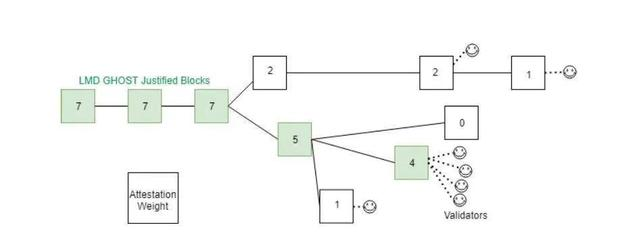Casper FFG

Casper FFG 全称为「Casper the Friendly Finality Gadget (Casper 友好的最终性小工具)」 ，是 Vitalik 提出的一个 PoW/PoS 混合的算法，目的是使 Ethereum 平滑过渡到纯PoS。

Vitalik 总结了四条规则，任何违反此四条规则的行为都要被取走押金。

• 提交（commit_req）：收到 2/3 节点的预备讯息后才能提交。
• 预备（prepare_req）：每个预备讯息只能指向某个也具有 2/3 节点预备讯息的高度（Epoch），且这些预备讯息也必须都指向同一个高度。
• 预备提交一致性（prepare_commit_consistency）：任何新的预备讯息只能指向最后一个已提交的或其他比其更新的高度。
• 不重复预备（no_double_prepare）：不能在同一个高度送出两次预备。

, 且使下列任一条件成立:

1. h(t1) = h(t2)

2. h(s1)

Casper FFG 运作

Casper FFG 通过检查点（Checkpoint）的合理化（Justified）和敲定（Finalized)）来完成共识。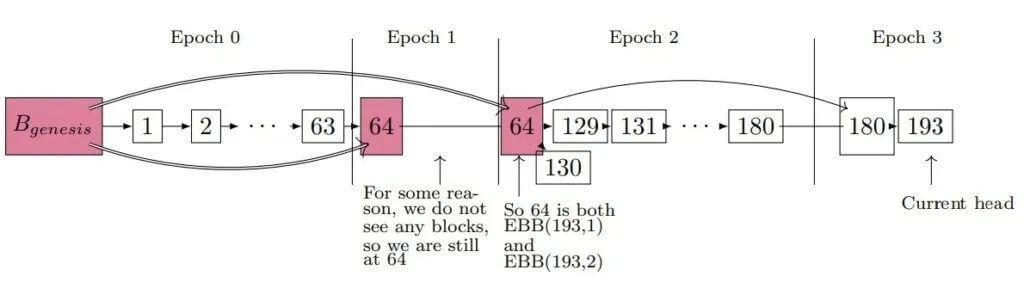• ⻅证被包含在最新的区块中
• ⻅证中包括了正确的检查点的投票
• ⻅证中包含了正确的最新区块
• ⻅证被很快地包含到链上
• ⻅证包含正确的分片区块（Phase1阶段实现）
``````def get_attestation_deltas(state: BeaconState) -> Tuple[Sequence[Gwei], Sequence[Gwei]]:      """     Return attestation reward/penalty deltas for each validator.     """      source_rewards, source_penalties = get_source_deltas(state)  # 计算检查点source奖惩      target_rewards, target_penalties = get_target_deltas(state)  # 计算检查点target奖惩      head_rewards, head_penalties = get_head_deltas(state)  # 计算最新区块奖惩      inclusion_delay_rewards, _ = get_inclusion_delay_deltas(state)  # 计算入块延迟奖惩      _, inactivity_penalties = get_inactivity_penalty_deltas(state)
rewards = [ source_rewards[i] + target_rewards[i] + head_rewards[i] + inclusion_delay_rewards[i] for i in range(len(state.validators)) ]
penalties = [ source_penalties[i] + target_penalties[i] + head_penalties[i] + inactivity_penalties[i] for i in range(len(state.validators)) ]
return rewards, penalties``````

（向左滑动，查看完整代码）

``def get_attestation_component_deltas(state: BeaconState,                                       attestations: Sequence[PendingAttestation]                                       ) -> Tuple[Sequence[Gwei], Sequence[Gwei]]:      """     Helper with shared logic for use by get source, target, and head deltas functions     """      rewards = [Gwei(0)] * len(state.validators)      penalties = [Gwei(0)] * len(state.validators)      total_balance = get_total_active_balance(state)      unslashed_attesting_indices = get_unslashed_attesting_indices(state, attestations)      attesting_balance = get_total_balance(state, unslashed_attesting_indices)      for index in get_eligible_validator_indices(state):          if index in unslashed_attesting_indices:              increment = EFFECTIVE_BALANCE_INCREMENT  # Factored out from balance totals to avoid uint64 overflow              if is_in_inactivity_leak(state):                  # Since full base reward will be canceled out by inactivity penalty deltas,                  # optimal participation receives full base reward compensation here.                  rewards[index] += get_base_reward(state, index)              else:                  reward_numerator = get_base_reward(state, index) * (attesting_balance // increment)                  rewards[index] += reward_numerator // (total_balance // increment)          else:              penalties[index] += get_base_reward(state, index) ``

（向左滑动，查看完整代码）

``reward_numerator = get_base_reward(state, index) * (attesting_balance // increment)   rewards[index] += reward_numerator // (total_balance // increment)  ``

（向左滑动，查看完整代码）

``def get_base_reward(state: BeaconState, index: ValidatorIndex) -> Gwei:      total_balance = get_total_active_balance(state)      effective_balance = state.validators[index].effective_balance      return Gwei(effective_balance * BASE_REWARD_FACTOR // integer_squareroot(total_balance) // BASE_REWARDS_PER_EPOCH)      # BASE_REWARD_FACTOR = 64 基础奖励倍数      # BASE_REWARDS_PER_EPOCH = 4 每个Epoch的基础奖励      # effective_balance 验证人的有效余额      # integer_squareroot(total_balance) 所有有效余额的开平方``

（向左滑动，查看完整代码）

``def get_proposer_reward(state: BeaconState, attesting_index: ValidatorIndex) -> Gwei:    return Gwei(get_base_reward(state, attesting_index) // PROPOSER_REWARD_QUOTIENT)``